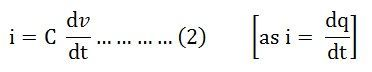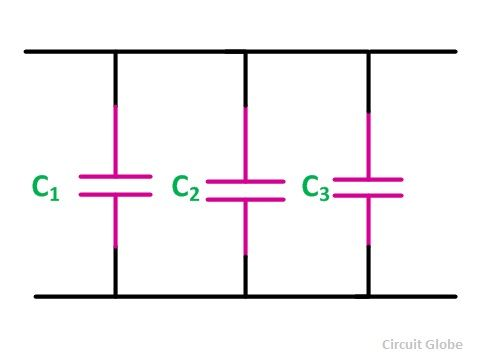# Capacitance

Capacitance is defined as the capability of an element to store electric charge. A capacitor store electric energy in the form of the electric field by the two electrodes of a capacitor, one as positive and other as negative.

In other words, capacitance is a measure of charge per unit voltage that can be stored in an element. It is denoted by (C), and its unit is Farad (F).

Contents:

The capacitance is mainly classified into two types; they are the self-capacitance and the mutual capacitance. The substance which has more self-capacitance store more electric charges and substance which have low capacitance stores less electrical charges.

## Explanation and Derivation of Capacitance

If the two parallel plates are connected, overlapping each other and are connected to a DC supply voltage as shown in the figure. The two plates are separated by an insulating dielectric so that the charge does not cross each other. One terminal of the parallel plate is connected to the positive supply and other to the negative supply. As the supply is ON, the capacitor starts charging, and it stores energy even if the supply is OFF.The capacitance equation is given by:Where,

• A is the overlapping area of the two plates in meter square
• d is the distance of separation between two plates in meters
• ε0 is known as the electric constant
• εr is the dielectric constant of the material between the two plates

The capacitance is said to be one farad if one coulomb of charge is stored with one volt across the two electrodes of the element. The element which has capacitance is called a capacitor.

The Charge on the capacitor at any instant of time isq is the amount of charge that can be stored in a capacitor of capacitance (C) against a potential difference of (v) volts.Where i, q and v represents the instantaneous value of current, charge and voltage respectively.Where

v0 is the initial voltage of the capacitor

vt is the final voltage of the capacitor

The power absorbed by the capacitor is given by the equation shown belowThe energy stored by the capacitor is given asThe current through the capacitor is zero if the applied voltage across the capacitor is constant.this means that when the DC voltage is applied across the capacitor with no initial charge, the capacitor first acts as a short circuit but as soon as it gets fully charged, the capacitor starts behaving as an open circuit.

The capacitor only stores energy and never dissipate the energy in any form. It can store a finite amount of energy, even if the current through the capacitor is zero.

Types of capacitor

The various types of capacitor are as follows:

• Paper Capacitor
• Air capacitor
• Plastic Capacitor
• Silver mica capacitor
• Ceramic capacitor
• Electrolytic capacitor
• Porcelain capacitor

## Series and Parallel Capacitance in a Circuit

### Series Capacitor Circuit

If the number of capacitors, for example, C1, C2, C3…..connected together in a series is called a series capacitor circuit. The current flowing in this type of circuit will be the same across all the capacitors as they are connected in series. The series connection of the capacitor is shown below in the figure:The equivalent capacitance is given by the equation as:

### Parallel Capacitor Circuit

If the number of capacitors is connected to each other as in parallel connection, the circuit is said to be a parallel capacitor circuit. The circuit is shown below:The equivalent capacitance in a parallel circuit is given by the equation shown below: